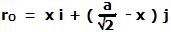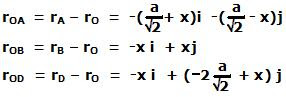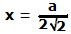## Tuesday, November 11, 2008

### Irodov Problem 1.237The three forces acting at A, B and D are given by,The net force acting on the square is given by,What the question essentially asks is that if you were asked to replace the three forces acting on square by a single force of magnitude Fnet, then at what point on the side BC, O would it be acting to create the same effect as the three forces?

If Fnet = 2Fi were acting at this point O, then the torque on the square about point O must be exactly 0, since the moment arm of Fnet is 0 about point O. In other words, we have to find a point O on side BC about which the net torque due to all the three forces is 0.

Suppose that the length of the side of the square is a. Let the origin (0,0) be at the center of the square. Then the coordinates of the corners A, B and D will be,The equation of the line BC is. Based on this, let the coordinate of O be,Then, we can compute the position vectors of point A, B and D with respect to O as,The net torque due to all the three forces about point O is given by,If the net torque about point O is to be 0 then, setting No = 0 we have. This corresponds to the mid point of the line BC.